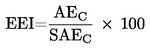## What is the Energy Efficiency Index (EEI)

The Energy Efficiency Index (EEI) is an index that shows how much resources are spent by an appliance to perform its functions. In the European Union, washing machines are assigned an energy efficiency class from A+++ to D. The definition of the class is based on the Energy Efficiency Index (EEI), which calculates the actual energy consumption of a washing machine and sets the washing machine’s energy efficiency class.
What the Energy Efficiency Index is, is the ratio of real consumption of resources to calculated consumption multiplied by 100.

The energy efficiency index is the percentage of real consumption of energy resources from the calculated one; if the washing machine consumes less than 46% of the calculated energy resources, it is assigned the A+++ index.

## Energy efficiency class Washing machine energy efficiency index

A +++ (most effective) EEI <46
A ++ 46 ≤ EEI <52
A + 52 ≤ EEI <59
A 59 ≤ EEI <68
IN 68 ≤ EEI <77
FROM 77 ≤ EEI <87
D (least effective) EEI ≥ 87

## Formula for calculating the Energy Efficiency Index (EEI)

The Energy Efficiency Index is calculated using the formula given above.

• AEc – The annual energy consumption of a household washing machine, determined by laboratory testing under real conditions.
• SAEc – The standard energy consumption of a washing machine is calculated using formulas, the calculation procedure is approved by EU directives.

In calculating the standard energy consumption of a washing machine, several types of formulas are used, the basic principle being as follows.

• the stand-by power consumption is taken into account, the example washing has ended but the laundry has not been removed and the washing machine is on standby.
• to calculate, it is accepted that one washing machine does 220 laundries per year and the clothes material is cotton. Of these 220 washes, 94 were washes at full load and a washing temperature of 60°C, 63 werehes at half load and a washing temperature of 60°C, 63 werehes at half load and a washing temperature of 40°C.
• laundry time is also taken into account.

These calculation formulas give very average data on energy efficiency. The error in these calculations is very high and can reach 40%. There is therefore little point in looking at the energy efficiency factor.

## Factors that may influence the calculation of the Energy Efficiency Index (EEI)

• Most of the electricity is used to heat water, the less water the machine uses, the sooner it heats it up and spends less electricity, the higher the class automatically, but the quality of the laundry is in question.
• washing machines with small tanks and a 4kg load capacity always have an A++ or A+++ class. A washing machine like this is more economical than a 6kg washing machine, but to wash 12kg of clothes, a 4kg machine will do 3 laundry jobs and a 6kg machine will only do 2 laundry jobs.
• The manufacturers do not specify how much water is used at half load, but one washing machine spends 50% of its full load and another 60%, this parameter is not described in the specifications.

It would be more correct to calculate how much energy is wasted on washing 1kg of laundry of the same cotton. But in this case, almost all washing machines will show very modest results.

## Summary of the Energy Efficiency Index (EEI)

The Energy Efficiency Index is a very ethereal value, and in reality almost does not reflect actual resource consumption. Many manufacturers somehow calculate this index, but it is very common to find that the same washing machine model with only design differences has different energy efficiency indices.